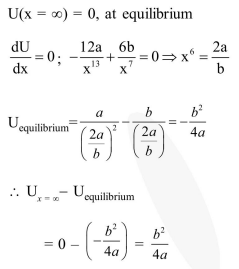# The potential energy function

Question:

The potential energy function for the force between two atoms in a diatomic molecule is approximately given by $U(x)=\frac{a}{x^{12}}-\frac{b}{x^{6}}$, where $a$ and $b$ are constant and $x$ is the distance between the atoms. if the dissociation energy of the molecule is $\mathrm{D}=\left[\mathrm{U}(\mathrm{x}=\infty)-\mathrm{U}_{\mathrm{at}}\right.$ equilibrium $]$, $\mathrm{D}$ is :

1. $\frac{b^{2}}{6 a}$

2. $\frac{b^{2}}{2 a}$

3. $\frac{b^{2}}{12 a}$

4. $\frac{b^{2}}{4 a}$

Correct Option: , 4

Solution: